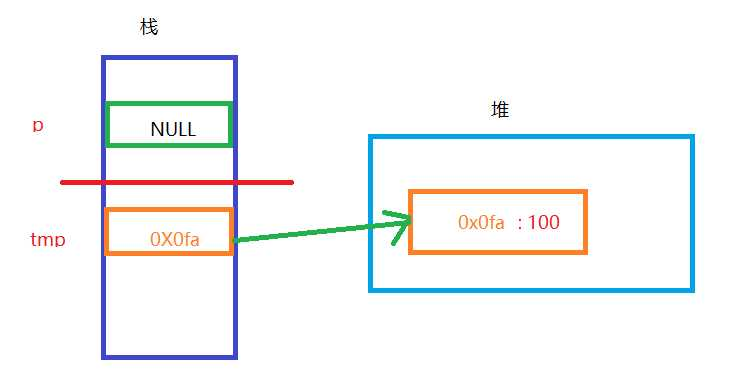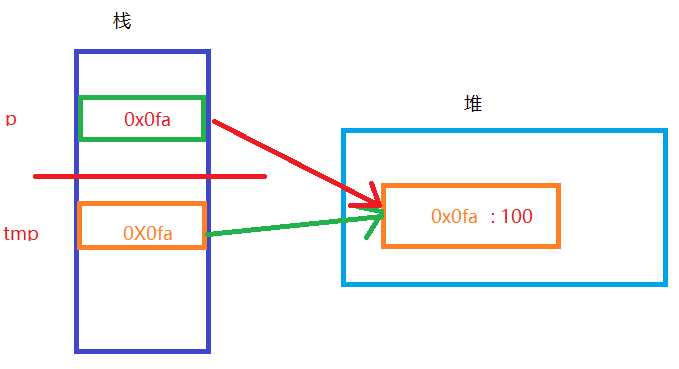# 1、返回栈中局部变量的地址

```#include <stdio.h>

int *fun(){
int a = 10; // a为当前方法栈中的局部变量，结束即释放
return &a;  // 所以传递出去的会是一个无效（非法）地址
}

int main(int argc, char const *argv[])
{
int *a = NULL;
a = fun();
*a = 100;   // error，操作非法内存
printf("a = %d\n", *a);
return 0;
}```

# 2、返回data区的地址

```#include <stdio.h>

int *fun(){
static int a = 10; // 静态局部变量，不会随着方法栈自动释放
return &a; // 返回的是有效地址
}

int main(int argc, char const *argv[])
{
int *a = NULL;
a = fun();
*a = 100;   // ok
printf("a = %d\n", *a);
return 0;
}```

# 3、指针作为形参传递（一）

```#include <stdio.h>
#include <stdlib.h>

void fun(int *tmp){
tmp = (int *)malloc(sizeof(int));   // 形参局部指针变量只在当前方法栈中有效
*tmp = 100;  // 当前栈中tmp动态分配的内存未释放，造成内存泄漏
}

int main(int argc, char const *argv[])
{
int *p = NULL;
fun(p); // p是空指针，没有指向，把p的值传给tmp
printf("*p = %d\n", *p);    // error 操作空指针所指向的内存
return 0;
}    ```# 4、指针作为形参传递（二）

```#include <stdio.h>
#include <stdlib.h>

void fun(int *tmp){
*tmp = 100;
}

int main(int argc, char const *argv[])
{
int *p = NULL;
p = (int *)malloc(sizeof(int));
fun(p); // 值传递
printf("*p = %d\n", *p);    // 100
return 0;
}```# 5、指针作为返回值

```#include <stdio.h>
#include <stdlib.h>

int * fun(){
int *tmp = NULL;
tmp = (int *)malloc(sizeof(int));   // 在堆中分配内存
*tmp = 100;                         // 堆内存赋值
return tmp;                         // 返回堆区地址，函数调用完毕不释放
}

int main(int argc, char const *argv[])
{
int *p = NULL;
p = fun(p);
printf("*p = %d\n", *p);    // 100

// free ：堆区空间需要手动释放
if (p != NULL) {
free(p);
p = NULL;
}

return 0;
}```

(0)
(0)© 2014 mamicode.com 版权所有 京ICP备13008772号-2